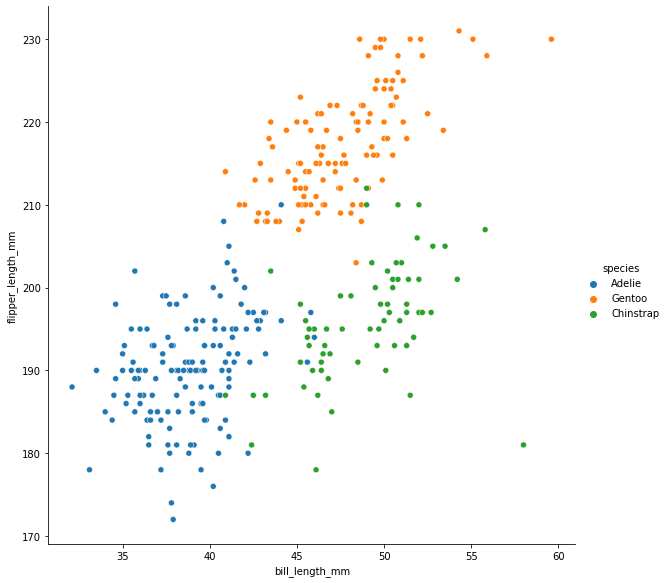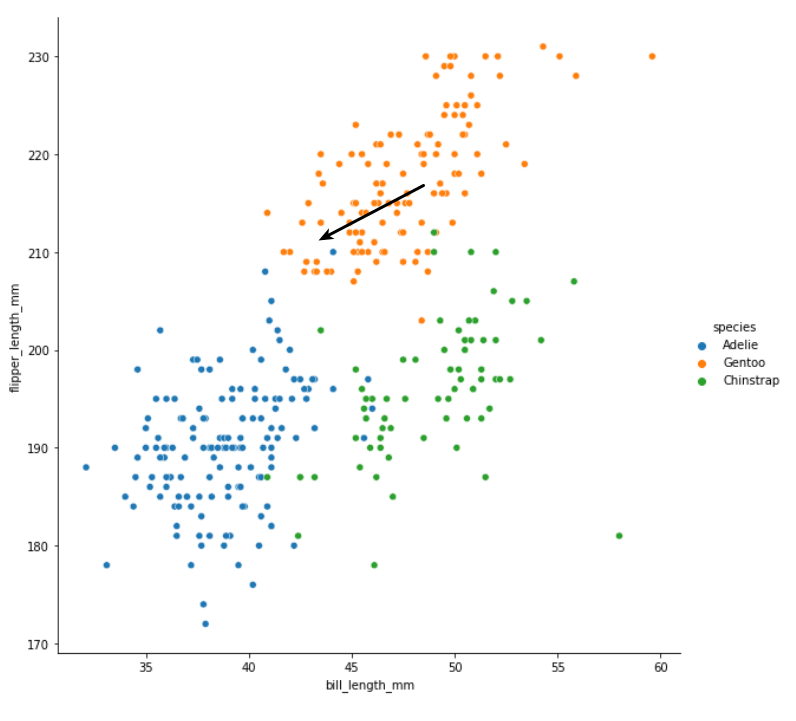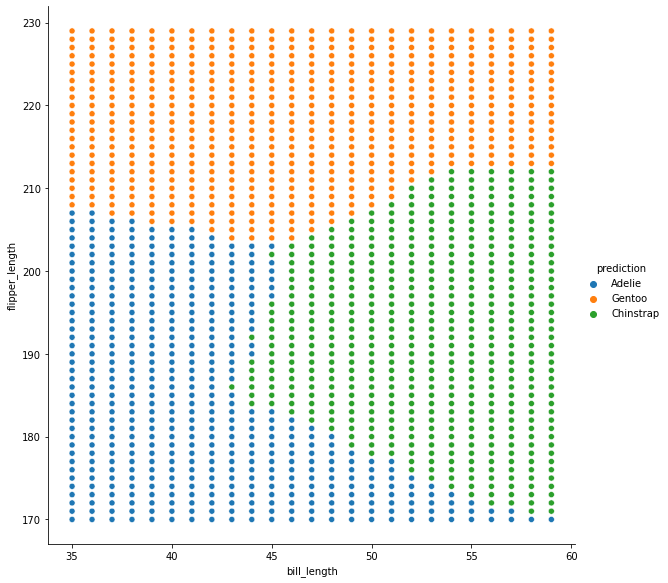# Machine learning for biology part two

## Introduction¶

In part one, we introduced the idea of programming by example, but didn't actually implement it. Instead we took an example of a classification problem - identifying penguin species based on their measurements:

In :
import pandas as pd

df = (
"https://raw.githubusercontent.com/allisonhorst/palmerpenguins/master/inst/extdata/penguins.csv",
)
.dropna() # missing data will confuse things
)[['species', 'bill_length_mm', 'flipper_length_mm']]

import seaborn as sns

sns.relplot(
data = df,
x = 'bill_length_mm',
y = 'flipper_length_mm',
hue = 'species',
height=8,
)

Out:
<seaborn.axisgrid.FacetGrid at 0x7f03cc7edfd0>and manually wrote a function to do the classification:

In :
def classify_penguin(bill_length, flipper_length):
if flipper_length > 205:
return 'Gentoo'
elif flipper_length <= 205 and bill_length > 45:
return 'Chinstrap'
else:


Looking at the above function, we might notice that the code is extremely specific to this problem - it will not help us if we have a different number of species, different measurements, or different distributions of classes.

## A more general approach¶

It would be nice to have a more general solution that we could apply to any classification problem. Let's begin by doing a little manual classification experiment. Pretend we have measured another penguin and we don't know the species. We'll add this new penguin to the chart and try to guess the species:In the above chart, the black arrow is pointing to the measurements of the new penguin - imagine there is another marker right at the tip of the arrow, and we have to decide which colour it belongs to.

Do you have your answer? I think that most people would intuitively say that this new penguin is a Gentoo penguin i.e. it belongs to the orange cluster. If asked to explain our choice, we might point out that most of the points closest to our new point are orange. A couple are blue, but it makes perfect sense here to go with the majority.

Let's try to turn this intuitive line of reasoning into a set of steps that we can implement as code. When we have a new point, we should:

• calculate the distance to all of the other points
• find the other points that are closest
• count up how many of the closest points belong to each species
• guess that our new point belongs to whichever species there is most of

### Onto some code¶

Now for the code: we can do this step by step using pandas. For an example, we will say that our new penguin has a bill length of 43mm and a flipper length of 211mm.

The most tricky step is calculating the distance. We can easily calcuate the difference in flipper length between each existing point and our new one:

In :
flipper_length_difference = df['flipper_length_mm'] - 211
flipper_length_difference

Out:
0     -30.0
1     -25.0
2     -16.0
4     -18.0
5     -21.0
...
339    -4.0
340    -9.0
341   -18.0
342    -1.0
343   -13.0
Name: flipper_length_mm, Length: 333, dtype: float64

and do the same for bill length:

In :
bill_length_difference = df['bill_length_mm'] - 45
bill_length_difference

Out:
0      -5.9
1      -5.5
2      -4.7
4      -8.3
5      -5.7
...
339    10.8
340    -1.5
341     4.6
342     5.8
343     5.2
Name: bill_length_mm, Length: 333, dtype: float64

For an explanation of the pandas magic that makes it possible to operate on complete columns in a single expression, see chapter 3 of the Biological exploration book.

Notice that in both outputs we have a mixture of positive and negative numbers. To find the overall distance between our new point and each of the existing ones, we can use Pythagoras. We square each distance, add them together, then take the square root of the result:

In :
# we need numpy's sqrt function to operate on complete columns
import numpy as np

overall_distance = np.sqrt(          # we want the square root of...
flipper_length_difference ** 2   # the square of the flipper distance
+ bill_length_difference ** 2    # plus the square of the bill distance
)
overall_distance

Out:
0      30.574663
1      25.597851
2      16.676031
4      19.821453
5      21.759825
...
339    11.516944
340     9.124144
341    18.578482
342     5.885576
343    14.001428
Length: 333, dtype: float64

For convenience, we will add this as a new column to our dataframe:

In :
df['distance to new point'] = overall_distance
df

Out:
species bill_length_mm flipper_length_mm distance to new point
... ... ... ... ...
339 Chinstrap 55.8 207.0 11.516944
340 Chinstrap 43.5 202.0 9.124144
341 Chinstrap 49.6 193.0 18.578482
342 Chinstrap 50.8 210.0 5.885576
343 Chinstrap 50.2 198.0 14.001428

333 rows × 4 columns

Now we can sort our dataframe by this new column:

In :
df.sort_values('distance to new point')

Out:
species bill_length_mm flipper_length_mm distance to new point
158 Gentoo 45.4 211.0 0.400000
204 Gentoo 45.1 210.0 1.004988
236 Gentoo 44.9 212.0 1.004988
274 Gentoo 45.2 212.0 1.019804
194 Gentoo 45.3 210.0 1.044031
... ... ... ... ...

333 rows × 4 columns

Notice how the points that end up at the top of the sorted table have measurements very close to our new point (45mm and 211mm). Using head we can select just the closest points - for now let's arbitrarily say that we want the ten closest:

In :
df.sort_values('distance to new point').head(10)

Out:
species bill_length_mm flipper_length_mm distance to new point
158 Gentoo 45.4 211.0 0.400000
204 Gentoo 45.1 210.0 1.004988
236 Gentoo 44.9 212.0 1.004988
274 Gentoo 45.2 212.0 1.019804
194 Gentoo 45.3 210.0 1.044031
152 Gentoo 46.1 211.0 1.100000
244 Gentoo 45.5 212.0 1.118034
198 Gentoo 45.5 210.0 1.118034
166 Gentoo 45.8 210.0 1.280625

Now we can easily see by looking at the first column that we have nine Gentoo penguins and one Adelie penguin among our closest points. But let's do this final step in code too:

In :
(
df.sort_values('distance to new point') # sort by distance
['species']                             # get the species column
.mode()                              # find the most common
)

Out:
'Gentoo'

Note that we need mode() in the last step because the mode method returns a series, as there might be multiple values that are equally common. We can imagine various different ways of breaking a tie, but for now we will just pick the first.

### And turning this into a function¶

Taking all of these steps together, we can turn them into a function that will start with a bill length and a flipper length, and return the guess for the species:

In :
def guess_species(bill_length, flipper_length):

# calculate distances and add to the dataframe
flipper_length_difference = df['flipper_length_mm'] - flipper_length
bill_length_difference = df['bill_length_mm'] - bill_length
overall_distance = np.sqrt(
flipper_length_difference ** 2
+ bill_length_difference ** 2
)
df['distance to new point'] = overall_distance

# find closest points and calculate most common species
most_common_species = (
df.sort_values('distance to new point')
['species']
.mode()
)

# the most common species is our guess
return most_common_species


This function looks kind of complicated, but it's just implementing the same rules that we humans follow intuitively. Let's check that if we put our original new point in we get the same output:

In :
guess_species(45, 211)

Out:
'Gentoo'

Of course, we can try some other points now as well:

In :
guess_species(40, 190)

Out:
'Adelie'

and get different outputs.

## Exploring the new function¶

Now that we have a function where we put in a pair of measurements and get out a species prediction, there are a number of interesting things that we can do with it.

### Testing the function¶

One thing we can do is to run the prediction function for each of the real penguin points:

In :
guesses = df.apply(
lambda p :
guess_species(p['bill_length_mm'], p['flipper_length_mm'])
, axis=1
)
guesses

Out:
0         Adelie
...
339    Chinstrap
341    Chinstrap
342       Gentoo
343    Chinstrap
Length: 333, dtype: object

and compare the guesses to the true species:

In :
(guesses == df['species']).value_counts(normalize=True)

Out:
True     0.951952
False    0.048048
dtype: float64

Chapter 13 of the Biological exploration book has more details on how apply works.

At first glance our function seems to be performing quite well, guessing right 95% of the time - but we will come back to this point later!

### Exploring the prediction landscape¶

Another thing that we can do is run our function on many different pairs of made up measurements and visualise the results. For example we can take all the bill lengths between 35mm and 60mm:

In :
bill_lengths = np.arange(35, 60)
bill_lengths

Out:
array([35, 36, 37, 38, 39, 40, 41, 42, 43, 44, 45, 46, 47, 48, 49, 50, 51,
52, 53, 54, 55, 56, 57, 58, 59])

and all the flipper lengths between 170mm and 230mm:

In :
flipper_lengths = np.arange(170, 230)
flipper_lengths

Out:
array([170, 171, 172, 173, 174, 175, 176, 177, 178, 179, 180, 181, 182,
183, 184, 185, 186, 187, 188, 189, 190, 191, 192, 193, 194, 195,
196, 197, 198, 199, 200, 201, 202, 203, 204, 205, 206, 207, 208,
209, 210, 211, 212, 213, 214, 215, 216, 217, 218, 219, 220, 221,
222, 223, 224, 225, 226, 227, 228, 229])

and plug each combination into our function to generate a guess:

In :
for bill_length in bill_lengths:
for flipper_length in flipper_lengths:
guess = guess_species(bill_length, flipper_length)


These data will be easiest to work with if we turn them into a dataframe:

In :
data = []
for bill_length in bill_lengths:
for flipper_length in flipper_lengths:
guess = guess_species(bill_length, flipper_length)
data.append((bill_length, flipper_length, guess))

data = pd.DataFrame(data, columns=['bill_length', 'flipper_length', 'prediction'])
data

Out:
bill_length flipper_length prediction
... ... ... ...
1495 59 225 Gentoo
1496 59 226 Gentoo
1497 59 227 Gentoo
1498 59 228 Gentoo
1499 59 229 Gentoo

1500 rows × 3 columns

Now see what happens if we plot these made-up points using the same code we used to plot the real points:

In :
sns.relplot(
data = data,
x = 'bill_length',
y = 'flipper_length',
hue = 'prediction',
height=8,
)

Out:
<seaborn.axisgrid.FacetGrid at 0x7f03cccfba90>We see how the evenly spaced grid of points shows us what the prediction would be for a new point that falls in various parts of the chart.

Here's the same chart but with a more tightly spaced grid (going up in steps of 0.2mm rather than 1mm):

In :
bill_lengths = np.arange(35, 60, 0.2)
flipper_lengths = np.arange(170, 230, 0.2)

data = []
for bill_length in bill_lengths:
for flipper_length in flipper_lengths:
guess = guess_species(bill_length, flipper_length)
data.append((bill_length, flipper_length, guess))

data = pd.DataFrame(data, columns=['bill_length', 'flipper_length', 'prediction'])

sns.relplot(
data = data,
x = 'bill_length',
y = 'flipper_length',
hue = 'prediction',
height=8,

<seaborn.axisgrid.FacetGrid at 0x7f03c9b36910>